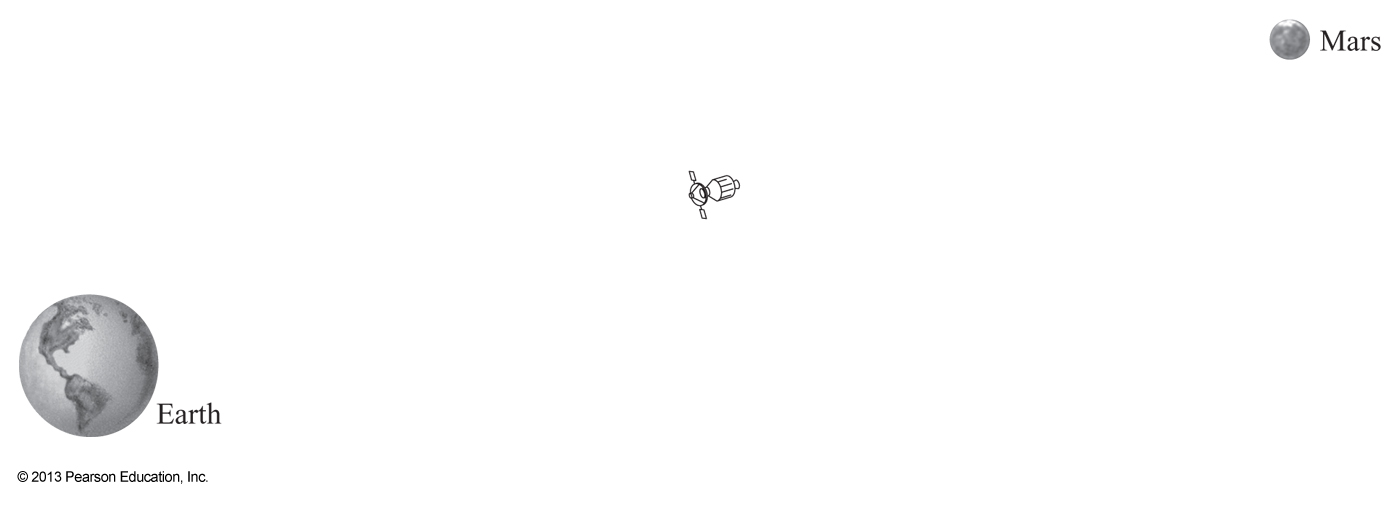5/5

# Browse Over 3 Million Questions

Let Our Professionals Assist You With Research and Writing.# Astronomy

## smartessays.net

Newton’s Law of Universal Gravitation is an equation:

## Forcedue to Gravity=GMassof the larger objectMassof the smaller objectDistancebetween objects2

G is the gravitational constant. For today, just assume it is 1 and you can ignore it. You can do this because it is simply a number and doesn’t affect the relationship between the other values.

Let’s examine the Force (due to Gravity) between the Earth (the larger object) and the Moon (the smaller object.

1. How would the Force (due to Gravity) between the Earth and the Moon change if the Moon were suddenly 2 times its current mass?
1. Would the Force (due to Gravity) increase or decrease?
2. By how many times?
2. How much Force (due to Gravity) is there between the Earth and the Moon?
1. How much Force (due to Gravity) does the Earth exert on the Moon? Fill in the blanks on this equation with words in order to answer this question.

## Forcedue to Gravity=GMass_________________________Mass__________________Distance_______________________2

1. How much Force due to Gravity does the Moon exert on the Earth? Fill in the blanks on this equation with words to answer this question.

## Forcedue to Gravity=GMass_________________________Mass__________________Distance_______________________2

1. How do these two compare?

The strength of the Force (due to Gravity) that the Earth exerts on the Moon

versus

The strength of the Force (due to Gravity) that the Moon exerts on the Earth

1. Are they the same or different?
2. Study the picture below. It is not to scale at all. This is a spacecraft travelling away from the Earth.1. Assume the spacecraft travels in a straight line away from the Earth. Label the point where the Force (due to Gravity) from the Earth on the spacecraft is the strongest.
2. Now label the point where the Force (due to Gravity) from the Earth on the spacecraft is the weakest.
3. Draw a point halfway between the Earth and the spacecraft, label that point A. Label the spacecraft’s current position as point B. The distance from the Earth to point A is 1. Label the distance from the Earth to point B as 2.
1. Fill out this equation for when the spacecraft is at point A. Use numbers for distance:

## Forcedue to Gravity=GMass_________________________Mass__________________Distance_______________________2

1. Fill out this equation for when the spacecraft is at point B:

## Forcedue to Gravity=GMass_________________________Mass__________________Distance_______________________2

1. Simplify the equations in parts 4a and 4b. You’ll end up making all variables that are the same equal to 1, and comparing just the difference. You should have Force=a fraction for both:
2. How would the Force (due to Gravity) between the Earth and the Moon change if the Moon were suddenly 2 times farther away?
1. Would the Force (due to Gravity) increase or decrease?
2. By how many times?
3. Study the picture below. It is not to scale at all. This is a spacecraft travelling from the Earth to Mars.1. Draw a straight line path between the Earth and Mars.
2. Remember that this spacecraft is halfway along its journey between the Earth and Mars. Where along that path would the Force (due to gravity) from Earth be strongest on the spacecraft?

Label this point on the diagram.

1. Where along that path would the Force (due to gravity) from Mars be strongest on the spacecraft?

Label this point on the diagram.

1. Now. Where along the path would the Force (due to gravity) on the spacecraft be balanced (equal) from Mars and from the Earth?

Label this point on the diagram.

1. When you finish that, write out the equations for the Force (due to gravity) on the spacecraft due to Mars and also due to Earth. Solve for distance. Remember that the distance to Earth is not the same as the distance to Mars.

##### “Looking for a Similar Assignment? Get Expert Help at an Amazing Discount!”Astronomy was first posted on July 24, 2019 at 4:43 pm.

## “Place This Order Or A Similar Order With Smart Essays Today And Get An Amazing Discount” (Coupon Code: SAVE15)

##### FREE FEATURES

FREE Reference page
FREE On-time Delivery
FREE Selection of Topics
FREE Unlimited Revisions# Affordable Writing Service

We guarantee a perfect price-quality balance to all students. The more pages you order, the less you pay.